Alternative schemes for advection attempt to overcome problems with boundedness and accuracy of the linear and upwind schemes respectively. Many schemes apply a limiter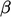between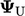from upwind and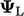from the linear scheme Eq. (3.4 ) according to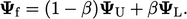(3.11)
When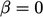, the scheme reduces to upwind and it becomes linear interpolation when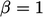. For a uniform mesh (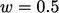),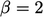represents interpolation using the downwind cell value.

Limited schemes attempt to optimiseat each face, based on the local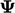, to maximise accuracy whilst maintaining boundedness.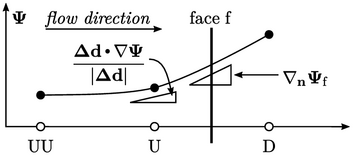Many schemes analyse the change in gradient of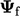between the face and upwind cell in the direction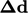connecting cell centres. They deﬁne a function of a ratio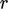of consecutive gradients as: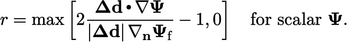(3.12)
There are numerous published schemes that deﬁne the limiter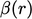as a function of the gradient ratio. Those schemes that are most useful are described in the following sections.

### Total variation diminishing schemes

Many useful schemes fall into a class known as Total Variation Diminishing (TVD).7 The TVD idea is that if the total variation of ﬁeld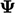does not increase in time, “overshoots” and oscillations associated with unboundedness will not occur.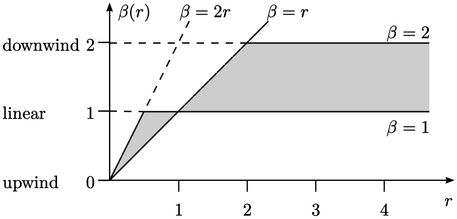To qualify as TVD, the limiter function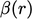must fall within the shaded area in a Sweby diagram (above).8 The TVD concept is a 1D analysis. For 3D CFD on irregular polyhedral meshes, oscillations are more likely to occur with TVD schemes whosefunctions tend signiﬁcantly to downwind, i.e. towards the upper part of the shaded area near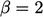.

A further property of a limited scheme is symmetry. A scheme is symmetric when the condition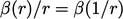is satisﬁed. When this occurs, the scheme applies the same limiter to the gradient of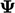, irrespective of the sign of its gradient. As a consequence, a property, initialised with a symmetric proﬁle, e.g. a bell curve, will retain its symmetry under advection.

7Ami Harten, High resolution schemes for hyperbolic conservation laws, 1983.
8Peter Sweby, High resolution schemes using ﬂux limiters for hyperbolic conservation laws, 1984.

Notes on CFD: General Principles - 3.11 Limited advection schemes